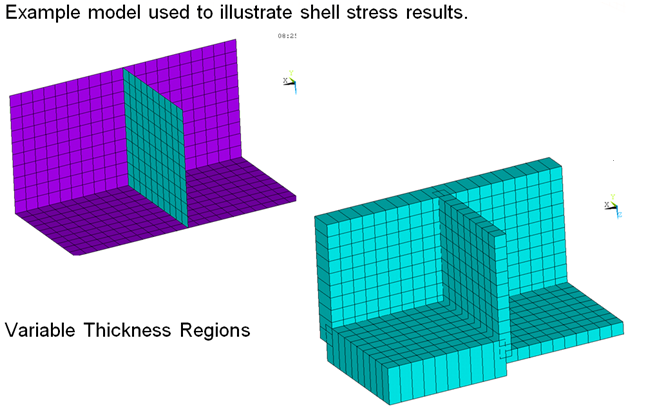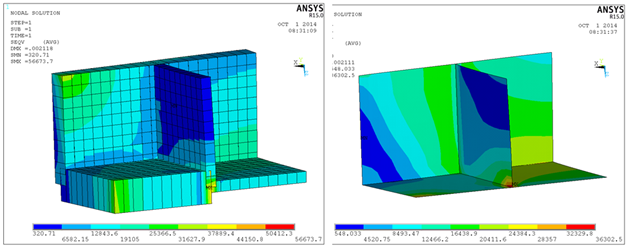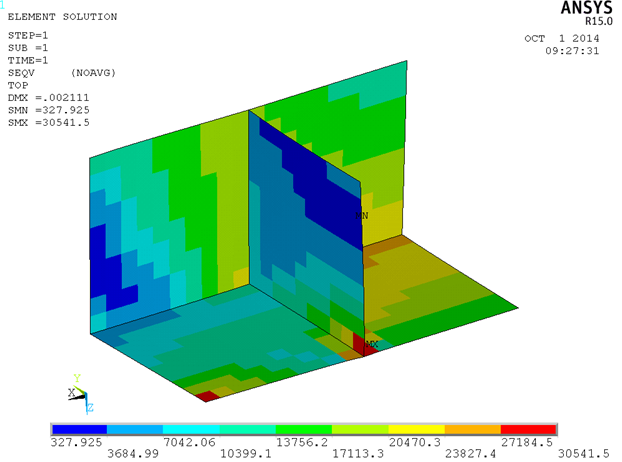you are here:/ News & Insights / Engineering Advantage Blog / Understanding and Using Shell Element Results - Part I

# Understanding and Using Shell Element Results - Part INovember 21, 2014 By: Peter Barrett

When performing a finite element analysis, shell elements can save a lot of computational time and yield accurate stress results when used properly to model structures whose thickness is small relative to the other dimensions. However, unlike solid elements where stress data is relatively straightforward to understand, the analyst must be careful to avoid a number of common pitfalls when interpreting stresses from shell elements.

The first questions any engineer should answer when postprocessing shells are: What do these results mean? and How are these results going to be used?  Items I will be investigating are listed below and explained in more detail through examples in Part I and Part II of this post.

• Are the stresses I plotted real and what are the limitations?
• Are only nominal stress results needed?
• How do I calculate local hot spot stresses needed for fatigue?
• If this a bolted or welded region that I am plotting stresses in, how do I use them?
• When should I use forces and moments instead of stresses?

The first two subjects will be addressed in this post, and items 3-5 will be addressed in Part II of this post, so stay tuned!!!

Figure 1 below illustrates a shell model of a local connection where each of these questions will be discussed.Depending upon the method deployed, stress plots for the above connection can yield a wide range of results with two extreme examples illustrated in Figure 2.  The peak stress on the left is predicted to be 57 ksi, while the peak stress on the right is 36 ksi for identical models.Are the stresses I plotted real and what are the limitations?

First, it should be noted that neither of the stress plots illustrated in Figure 2 capture an accurate result at the connection, since the shell model of the intersection between plates creates a singular condition.  Stresses are also approximate due to the mid-plane node sharing and overlapping of each plate at the joint. The overlap effect presents an inherent stiffness inaccuracy in the model. Sometimes this can be minimized by offsetting the shell nodes so that they are located at the shell top or bottom instead of the midplane.  The stress singularity issue will still remain, however.

For this case, the stresses on the left represent the condition where each plate is evaluated independently and stresses are plotted on both the top and bottom surfaces of the shells.  While these stresses are not accurate, they at least represent a stress calculation consistent with the independent plate geometries.  On the right, nodal stress averaging is done between plates of different thickness and direction, where the top surface stresses for each plate are averaged together.  This is garbage!  When plotting stresses in shells, isolating plates independently is preferred to avoid this non-physical averaging.  It is also important to consider whether the plot is illustrating the top and bottom (shown with the 3D shape), top-only, bottom-only or mid-plane stress. You should also verify that all the shell normals are consistent so that the top and bottom surfaces are the same for all elements.

Are only nominal stress results needed?

If the loading is not cyclic and the analysis goal is to compute plate/slab ultimate capacity then nominal stresses might be a preferred output.   For cases where nominal stresses are needed, local hot spot shell stresses can often be ignored since the real stresses will not be captured by the shell model anyway if the local connection geometry is not accounted for or if re-distribution upon local stress yielding or local concrete cracking occurs. Nominal stresses can often be evaluated using element centroid  stresses to reduce the singular stress influence.influence (See Figure 3 below for an example of centroidal top surface stresses). Since centroidal data averaging only occurs between the individual element integration points, the entire connection can be plotted without the fear of non-physical averaging.  It should be noted that top and bottom surfaces are typically evaluated with independent plots in this case. A relatively coarse mesh should be used with this approach. Click here for Part II, where l discuss calculating local hot spot stress, how to use bolted or welded regions, and when to use forces and moments.